Next: Simple Flight Problems Up: Incompressible Aerodynamics Previous: Aerodynamic Forces

# Ellipsoidal Airfoils

Consider an ellipsoidal airfoil whose outer surface is specified by the parametric equations(9.91)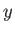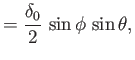(9.92)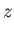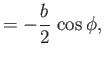(9.93)

where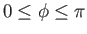and. Here,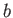is the wingspan,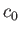the maximum wing width, and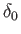the maximum wing thickness. Note that the wing's cross-section is elliptical both in the-and the-planes. It is assumed that: that is, the wingspan is greater than the wing width, which in turn is much greater than the wing thickness. At fixed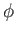(i.e., fixed), the width and thickness of the airfoil are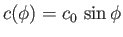and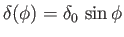, respectively.

Assuming that the two-dimensional result (9.44) holds at fixed, we deduce that the air circulation about the wing satisfies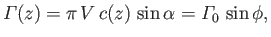(9.94)

where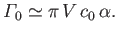(9.95)

Here, the angle of attack,, is assumed to be small. From Equations (9.90) and (9.94), the downwash velocity in the region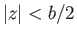is given by(9.96)

The integrand appearing in the integral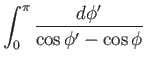(9.97)

is singular when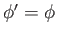. However, we can still obtain a finite value for the integral by taking its Cauchy principal part: that is,(9.98)

Physically, this is equivalent to omitting the contribution of the local free vortex at a given point on the airfoil's trailing edge to the downwash velocity induced at that point, which is reasonable because a vortex induces zero velocity at its center. Hence, we obtain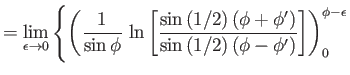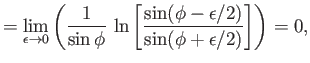(9.99)

which implies that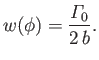(9.100)

In the region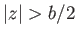, we can write, so that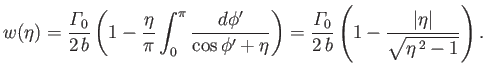(9.101)

Hence, we conclude that the downwash velocity profile induced by an ellipsoidal airfoil takes the form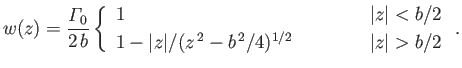(9.102)

This profile is shown in Figure 9.13. It can be seen that the downwash velocity is uniform and positive in the region between the wingtips (i.e.,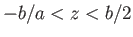), but negative and decaying in the region outside the wingtips. Hence, we conclude that as air passes over an airfoil subject to an upward lift it acquires a net downward velocity component, which, of course, is a consequence of the reaction to the lift. On the other hand, the air immediately behind and to the sides of the airfoil acquires a net upward velocity component. In other words, the lift acting on the airfoil is associated with a downwash of air directly behind, and an upwash behind and to either side of, the airfoil. The existence of upwash slightly behind and to the side of a flying object allows us to explain the V-formation adopted by wild geese--a bird flying in the upwash of another bird needs to generate less lift in order to stay in the air, and, consequently, experiences less induced drag.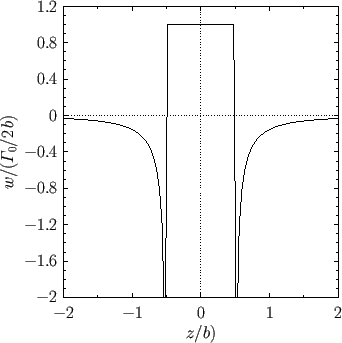It follows from Equation (9.93) and (9.94) that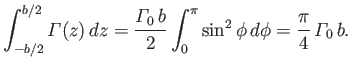(9.103)

Hence, Equation (9.80), (9.82), and (9.100) yield the following expression for the lift and induced drag acting on an ellipsoidal airfoil,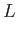(9.104)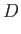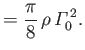(9.105)

The surface area of the airfoil in the-plane is(9.106)

Moreover, the airfoil's aspect-ratio is conventionally defined as the length to width ratio for a rectangle of lengththat has the same area as the airfoil: that is,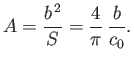(9.107)

It thus follows from Equation (9.95) that(9.108)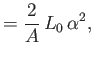(9.109)

where(9.110)Next: Simple Flight Problems Up: Incompressible Aerodynamics Previous: Aerodynamic Forces
Richard Fitzpatrick 2016-03-31